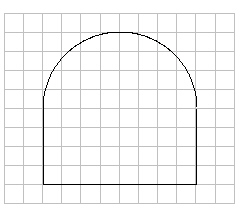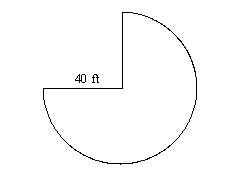Name:    Chapter 8 Test

Multiple Choice
Identify the choice that best completes the statement or answers the question.

Find the perimeter of the figure.

1.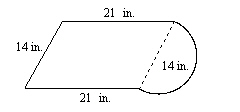a. 79.1 in. c. 121.94 in. b. 84 in. d. 78 in.

2.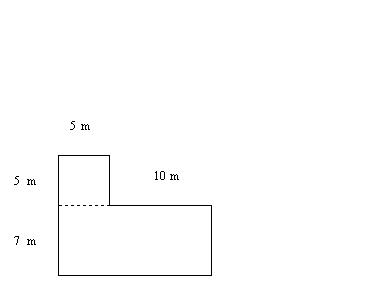a. 54 m c. 27 m b. 59 m d. 130 m

Find the area of the circle.  Use 3.14 or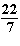for p.

3.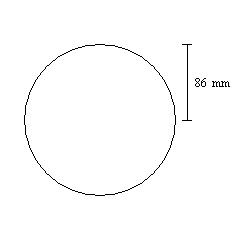a. about 540.08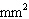c. about 23,224.44b. about 23,223.44d. about 5,805.864.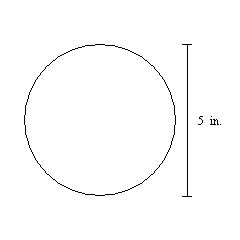a. about 20.735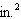c. about 78.5b. about 19.625d. about 15.7Find the area of the figure.

5.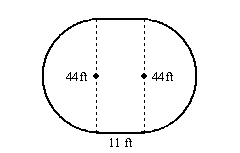a. 518.54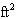c. 2003.76b. 622.16d. 2004.876.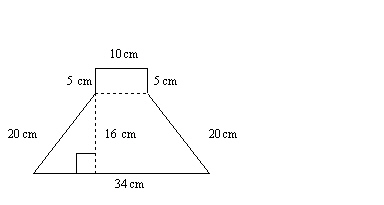a. 402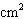c. 594b. 94d. 403.11Numeric Response

Find the radius of the circle.

1.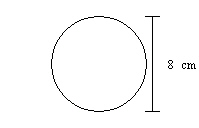2.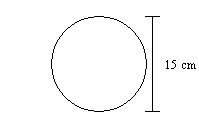Find the diameter of the circle.

3.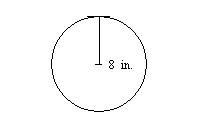4.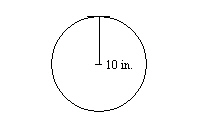Find the circumference of the circle. Use 3.14 or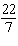for p.

5.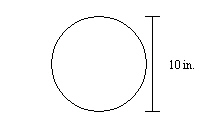6.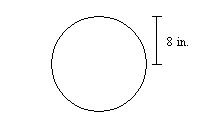Estimate the perimeter of the figure.

7.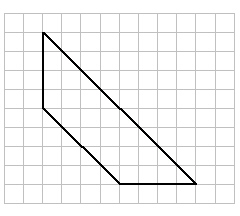Find the area of the figure.

8.